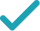Type a math problemSolve for xSteps Using the Quadratic Formula
Steps for Completing the Square
Swap sides so that all variable terms are on the left hand side.
Subtract from both sides.
This equation is in standard form: . Substitute for , for , and for in the quadratic formula, .
Square .
Multiply times .
Multiply times .
Take the square root of .
The opposite of is .
Multiply times .
Now solve the equation when is plus. Add to .
Divide by .
Now solve the equation when is minus. Subtract from .
Divide by .
The equation is now solved.
Solve for x (complex solution)Solve for yAssign yGraphGiving is as easy as 1, 2, 3
Get 1,000 points to donate to a school of your choice when you join Give With Bing
-2x^{2}-8x+9=y
Swap sides so that all variable terms are on the left hand side.
-2x^{2}-8x+9-y=0
Subtract y from both sides.
x=\frac{-\left(-8\right)±\sqrt{\left(-8\right)^{2}-4\left(-2\right)\left(9-y\right)}}{2\left(-2\right)}
This equation is in standard form: ax^{2}+bx+c=0. Substitute -2 for a, -8 for b, and 9-y for c in the quadratic formula, \frac{-b±\sqrt{b^{2}-4ac}}{2a}.
x=\frac{-\left(-8\right)±\sqrt{64-4\left(-2\right)\left(9-y\right)}}{2\left(-2\right)}
Square -8.
x=\frac{-\left(-8\right)±\sqrt{64+8\left(9-y\right)}}{2\left(-2\right)}
Multiply -4 times -2.
x=\frac{-\left(-8\right)±\sqrt{64+72-8y}}{2\left(-2\right)}
Multiply 8 times 9-y.
x=\frac{-\left(-8\right)±\sqrt{136-8y}}{2\left(-2\right)}
x=\frac{-\left(-8\right)±2\sqrt{34-2y}}{2\left(-2\right)}
Take the square root of 136-8y.
x=\frac{8±2\sqrt{34-2y}}{2\left(-2\right)}
The opposite of -8 is 8.
x=\frac{8±2\sqrt{34-2y}}{-4}
Multiply 2 times -2.
x=\frac{2\sqrt{34-2y}+8}{-4}
Now solve the equation x=\frac{8±2\sqrt{34-2y}}{-4} when ± is plus. Add 8 to 2\sqrt{34-2y}.
x=-\frac{\sqrt{34-2y}}{2}-2
Divide 8+2\sqrt{34-2y} by -4.
x=\frac{-2\sqrt{34-2y}+8}{-4}
Now solve the equation x=\frac{8±2\sqrt{34-2y}}{-4} when ± is minus. Subtract 2\sqrt{34-2y} from 8.
x=\frac{\sqrt{34-2y}}{2}-2
Divide 8-2\sqrt{34-2y} by -4.
x=-\frac{\sqrt{34-2y}}{2}-2 x=\frac{\sqrt{34-2y}}{2}-2
The equation is now solved.
-2x^{2}-8x+9=y
Swap sides so that all variable terms are on the left hand side.
-2x^{2}-8x=y-9
Subtract 9 from both sides.
\frac{-2x^{2}-8x}{-2}=\frac{y-9}{-2}
Divide both sides by -2.
x^{2}+\frac{-8}{-2}x=\frac{y-9}{-2}
Dividing by -2 undoes the multiplication by -2.
x^{2}+4x=\frac{y-9}{-2}
Divide -8 by -2.
x^{2}+4x=\frac{9-y}{2}
Divide y-9 by -2.
x^{2}+4x+2^{2}=\frac{9-y}{2}+2^{2}
Divide 4, the coefficient of the x term, by 2 to get 2. Then add the square of 2 to both sides of the equation. This step makes the left hand side of the equation a perfect square.
x^{2}+4x+4=\frac{9-y}{2}+4
Square 2.
x^{2}+4x+4=\frac{17-y}{2}